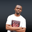Related Tags

# How to get values with the power of two using the Euphoria MethodNDUKWE CHIDERA K.

### Overview

The math library in Euphoria is very large and understandably so. Programs involve a lot of arithmetic evaluations* and *mathematical computations. Because of this, the Euphoria language comes with different math methods to execute several regular math operations.

In this shot, we shall take a look at a function that checks if the integer is a power of two or not.

### What is the powof2() function?

Before digging into this function, let’s understand what the power of 2 mathematically means.

A number is a power of 2 if that number can be achieved by exponentiating the integer 2 to any number ranging from zero to any number.

The powof2() method checks if the number provided can be achieved by exponentiating 2 to any value. This method can be used anywhere in our code. To do this, we add include std/math.e at the top end of our code.

### Syntax

powof2(value)


### Parameter

• value: This parameter can be an atom or a sequence. This means the value parameter is of the object data type. It is the value to be checked for the power of two.

### Return value

This function returns either 1 or 0. It returns 1 when the value checked is not a power of two.


include std/math.e

--start a for loop
for i = 1 to 16 do

--print the return value
? {i, powof2(i)}

--end the for loop
end for
Code exmaple of powof2() function

### Explanation

• Line 2: We include the math library.

• Line 4, 7 and 10: These are all comments.

• Line 5: A for loop is started and executes the action codes till the counter i— which starts from 1— is equal to 16.

• Line 8: We use the powof2() function and print the returned value to the screen.

RELATED TAGS

CONTRIBUTORNDUKWE CHIDERA K.
RELATED COURSES

View all Courses

Keep Exploring

Learn in-demand tech skills in half the time